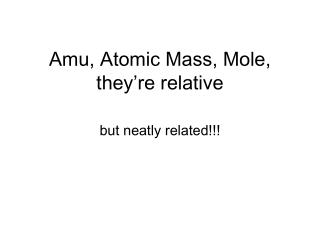DownloadDownload PresentationAmu, Atomic Mass, Mole, they’re relative

# Amu, Atomic Mass, Mole, they’re relative

Download Presentation## Amu, Atomic Mass, Mole, they’re relative

- - - - - - - - - - - - - - - - - - - - - - - - - - - E N D - - - - - - - - - - - - - - - - - - - - - - - - - - -
##### Presentation Transcript

1. Amu, Atomic Mass, Mole, they’re relative but neatly related!!!

2. In the early days how much does an element weigh? how much does an atom weigh? Just take a stab… “I decree that hydrogen will have a standard mass of 2!” Stanislao Cannizzaro (1826–1910),

3. Using Hydrogen as the relative standard • too small – • too much error • Think bigger – Oxygen sixteen! • it forms chemical compounds with many other elements, • lowest whole number and still have hydrogen > 1

4. Discovery of oxygen 17 and 18 – oops!! • no problem for the chemists • (1/16th of the average mass of oxygen) • Physicists, however, dealing with atoms required • a unit that distinguished between isotopes. • (1/16th of the mass of an atom of a particular isotope of oxygen)

5. In 1956, Alfred Nier (at the bar in the Hotel Krasnapolski in Amsterdam) suggested that the atomic weight scale be based on carbon-12.  • Physicists could care less • Chemists would have to adjust all their atomic • masses by 42 ppm!  • Six years later chemists and physicists agreed • on C12

6. Amadeo Avogadro • Italian physics professor • his law – “equal volumes of different gases at the same temperature and pressure contain equal numbers of atoms or molecules.”

7. Equal Volumes of gases O2 N2 Cl2 T = 0oC P = 1 atmosphere

8. Equal Number of Particles O2 N2 Cl2

9. but mass is not the same! O2 N2 Cl2 2 x 16g 2 x 14g 2 x 35g

10. Definition! A sample of any element with a mass equal to that element's atomic mass in grams contains one mole of atoms (6.02 x 1023 atoms) it’s also called the gram atomic mass or gam

11. Practice: • One mole of chromium weighs ______ grams. • One mole of chromium contains _______ atoms. • The gam of chromium is _________. • 0.5M of chromium weighs ________ grams. • 0.5M of chromium contains __________ atoms

12. Definition! A sample of any compound with a mass equal to that compound’s molecular mass (in grams) contains one mole of molecules (6.02 x 1023 molecules) It’s also called the gram molecular mass or gmm.

13. Practice: • One mole of water weighs ______ grams. • One mole of water contains _______ molecules. • One mole of water contains ______ atoms of H • One mole of water contains ______ atoms of O • The gmm of water is ________ • 0.2M of water weighs ________ grams. • 0.2M of water contains __________ molecules • 0.2M of water contains ______ grams of H • 0.2M of water contains ______ molecules of H

14. Definition! A sample of any ioniccompound with a mass equal to that compound’s unit formula mass (in grams) contains one mole of formula units (6.02 x 1023 molecules) It’s also called the gram formula mass or gfm.

15. Practice: • How many moles are there in 320 grams of CoCl2? • How many grams are there in 4.2M Ca(NO3) 2 ? • How many molecules are there in 4.2M Ca(NO3) 2 ? • 2.45 x 1023 molecules of CH4 are ______ moles?

16. Practice: • One mole of table salt weighs ______ grams. • One mole of table salt contains _______ formula units. • One mole of table salt contains ______ atoms of Na • One mole of table salt contains ______ atoms of Cl • 0.75M of NaCl weighs ________ grams. • 0.75M of NaCl contains __________ formula units

17. In Summary • Mass is a way to count numbers of particles • A mole represents 6.02 x 1023 particles, be they • atoms, molecules, formula units or whateva • The mass of 1 mole of a substance is = • 6.02 x 1023 particles x mass of one particle • for elementsgam = gram atomic mass • for molecular compounds gmm = gram molecular mass • for ionic compounds gfm = gram formula mass

18. Volume of 1 Mole of Gas STP = 0oC and 1 atmosphere One Mole of any gas occupies 22.4 liters at STP

19. Practice – Part I: • One mole of gaseous oxygen weighs ______ grams. • One mole of gaseous oxygen contains _______ molecules • One mole of gaseous oxygen occupies_______ liters • Practice – Part II: • 0.75M of gaseous oxygen weighs ________ grams. • 0.75M of gaseous oxygen contains __________ molecules. • 0.75M of gaseous oxygen occupies _______ liters at STP.